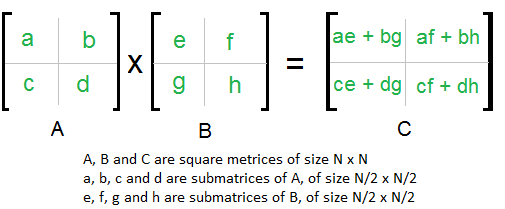# How to Perform Matrix Multiplication of given dimension in Python

In this Python tutorial, we will learn how to perform matrix multiplication in Python of any given dimension.

## MATRIX MULTIPLICATION in Python

Matrix multiplication is the multiplication of two matrices.

The necessary condition:

• R2(Number of Rows of the Second Matrix) = C1(Number of Columns of the First Matrix)
• Number of Rows of the Product Matrix =R1(Number of Rows of First Matrix)
• Number of Columns of the Product Matrix = C2(Number of Columns of the Second Matrix)From the above conditions and the image we can understand that:

`[A](m,n) X [B](n,s) = [P](m,s)`

where

• m is the number of rows of A
• n is the number of columns of A = number of rows of B
• s is the number of columns of B

You may also learn,

Let’s take a look at the code snippet.

## Python program for matrix multiplication

```r1=int(input("Enter number of Rows of Matrix A: "))
c1=int(input("Enter number of Columns of Matrix A: "))
A=[[0 for i in range(c1)] for j in range(r1)] #initialize matrix A
print("Enter Matrix Elements of A:")
#input matrix A
for i in range(r1):
for j in range(c1):
x=int(input())
A[i][j]=x
r2=int(input("Enter number of Rows of Matrix B: "))
c2=int(input("Enter number of Columns of Matrix B: "))
B=[[0 for i in range(c2)] for j in range(r2)] #initialize matrix B
print("Enter Matrix Elements of B:")
#input matrix B
for i in range(r2):
for j in range(c2):
x=int(input())
B[i][j]=x
if(c1==r2): #if no. of columns of matrix A is equal to no. of rows of matrix B
P=[[0 for i in range(c2)] for j in range(r1)] #initialize product matrix
for i in range(len(A)):
for j in range(c2):
for k in range(len(B)):
P[i][j]=P[i][j]+(A[i][k]*B[k][j]) #multiplication
#print the product matrix
print("Product of Matrices A and B: ")
for i in range(r1):
for j in range(c2):
print(P[i][j],end=" ")
print()
else: #if no. of columns of matrix A isn't equal to no. of rows of matrix B
print("Matrix Multiplication is not possible.")```

OUTPUT 1:

```Enter number of Rows of Matrix A: 3
Enter number of Columns of Matrix A: 3
Enter Matrix Elements of A:
1
2
3
4
5
6
7
8
9
Enter number of Rows of Matrix B: 3
Enter number of Columns of Matrix B: 2
Enter Matrix Elements of B:
2
4
6
8
1
3
Product of Matrices A and B:
17 29
44 74
71 119```

Here, the dimension of matrix A is 3X3. And the matrix B is of 3X2 dimension. Thus product matrix is 3X2.

OUTPUT 2:

```Enter number of Rows of Matrix A: 4
Enter number of Columns of Matrix A: 3
Enter Matrix Elements of A:
4
8
6
7
8
2
1
4
5
6
7
3
Enter number of Rows of Matrix B: 3
Enter number of Columns of Matrix B: 2
Enter Matrix Elements of B:
7
5
6
4
3
3
Product of Matrices A and B:
94 70
103 73
46 36
93 67```

The dimension of the Product matrix = (Number of rows of A) X (Number of columns of B)

Output 3:

```Enter number of Rows of Matrix A: 3
Enter number of Columns of Matrix A: 3
Enter Matrix Elements of A:
7
8
6
9
4
3
5
1
2
Enter number of Rows of Matrix B: 2
Enter number of Columns of Matrix B: 2
Enter Matrix Elements of B:
4
5
2
6
Matrix Multiplication is not possible.```

In this result, the number of rows of matrix B is not equal to the number of columns of matrix A. Thus the message shows that the matrix multiplication is not possible.